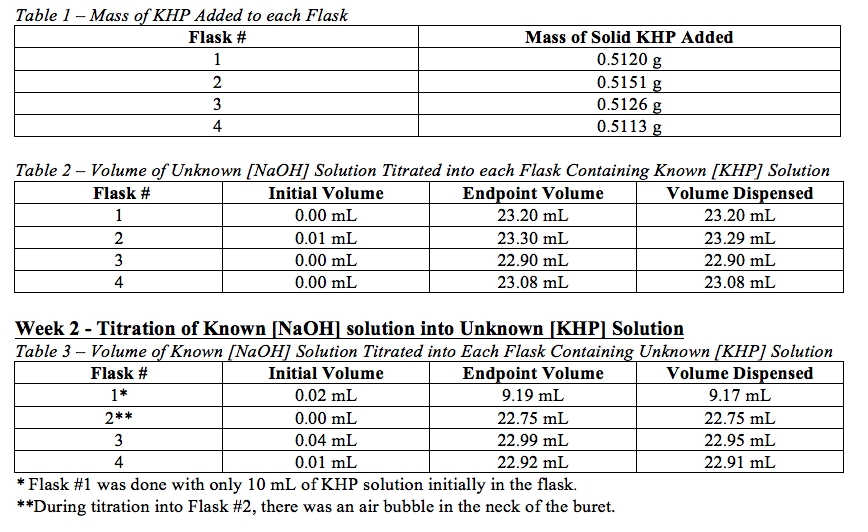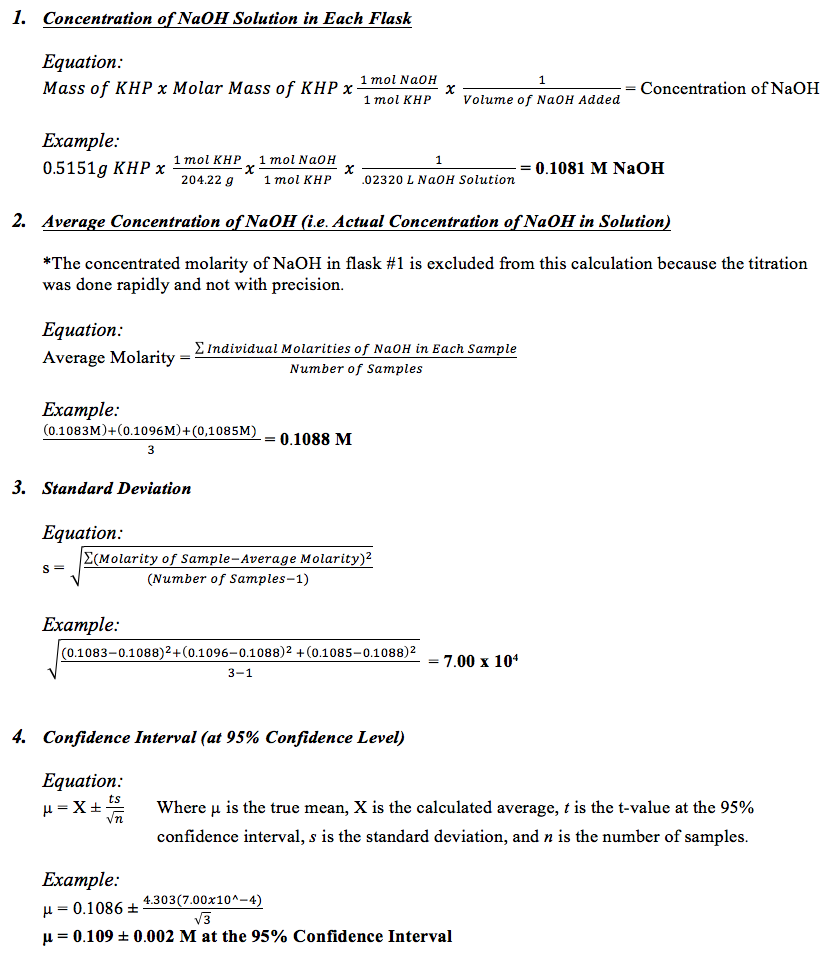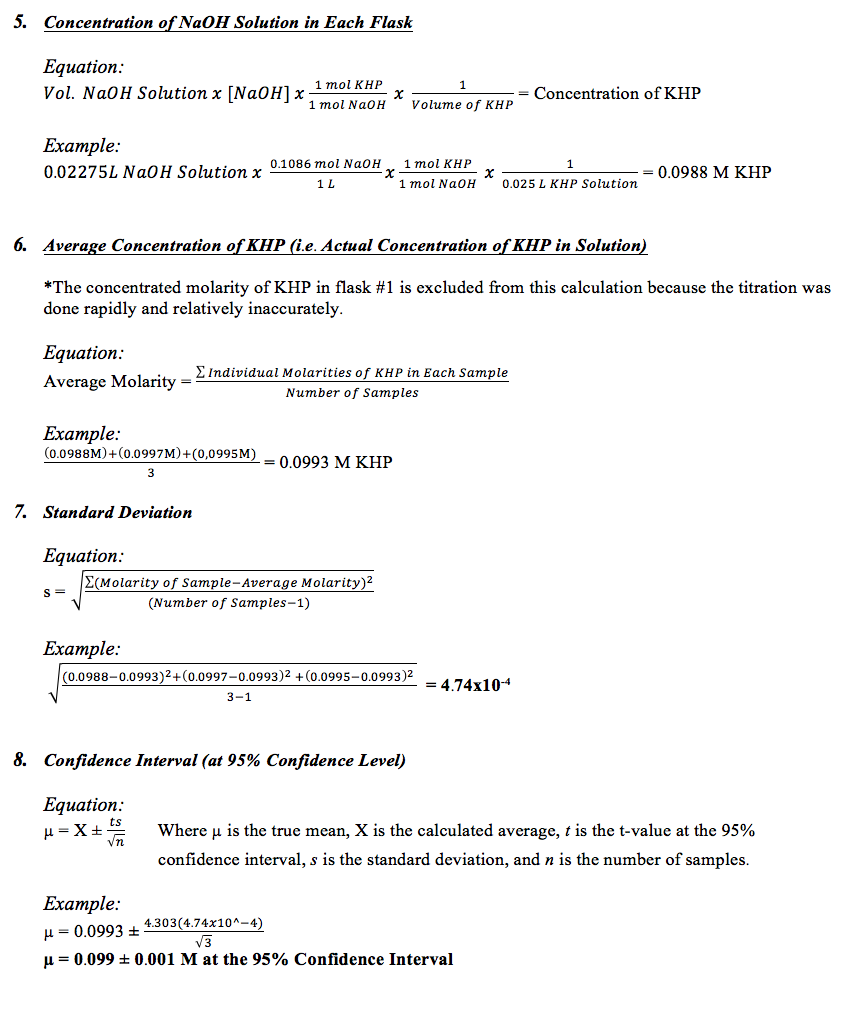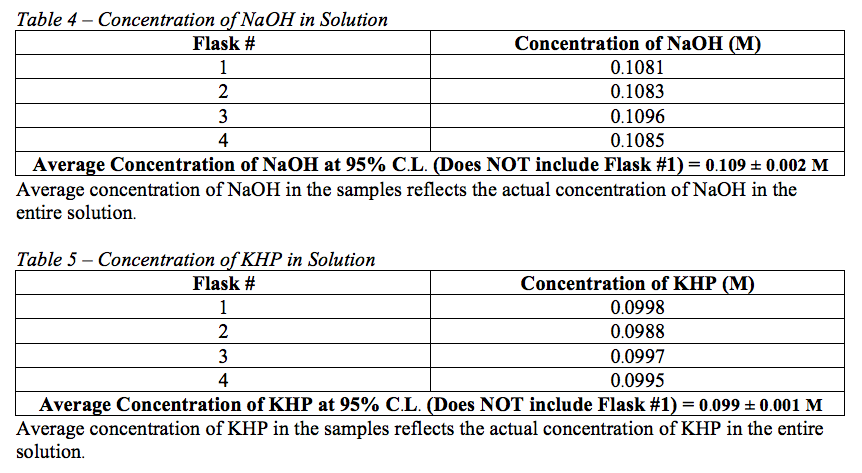# Preparing Standard Acid and Base

Abstract

Via acid/base titration with a primary standard (potassium hydrogen phthalate) an NaOH solution of unknown concentration was standardized. Once the NaOH solution was standardized, it was used as to determine the concentration of a KHP solution of unknown molarity.  In both instances, an acid/base indicator was used to signal the endpoint. Using the recorded volumes from the titrations, the molarity of the known solutions, and the molar mass of the compounds in the solutions of known concentration, the molarities NaOH and KHP in the solutions of unknown molarity were calculated. The molarity of NaOH in the unknown solution was established to be 0.109 ± 0.002 M at the 95% confidence level, and the concentration of the potassium hydrogen phthalate solution was determined to be 0.099 ± 0.001 M at the 95% confidence level.

Introduction

In the lab, sodium hydroxide and potassium hydrogen phthalate (KHP) are extensively used reagents. In order to utilize these reagents accurately, their exact concentrations must be determined. The purpose of this experiment was to create and standardize a solution of NaOH, and to then use that solution to determine the concentration of a solution containing an unknown molarity of KHP. NaOH solutions must be standardized because NaOH primary standard – the solution absorbs carbon dioxide from the atmosphere and reacts to form carbonate. In order to do this, a solution of approximately 0.1 M NaOH was created, and then titrated to the endpoint into a KHP solution of known concentration with phenolphthalein indicator. This titration was repeated four times, and the data from these titrations allowed the true concentration of the NaOH solution to be determined. The following week, the standardized NaOH solution was titrated to the endpoint into a KHP solution of unknown molarity with phenolphthalein indicator. This titration was also repeated four times, and the data from these titrations allowed the true concentration of the KHP solution to be established.

Experimental Information

Equipment

• 1-L beaker
• 1-L polyethylene bottle
• Class A 50-mL buret
• Magnetic stir bar
• Stir plate

Chemicals

• 50wt% NaOH
• Solid KHP
• Phenolphthalein indicator
• KHP solution of unknown molarity

Procedural Overview

Week 1: Boil 1L of distilled water for 5 minutes. Pour the water into a 1-L polyethylene bottle and add 5.33 mL 50wt% NaOH. Cap the bottle tightly, mix well, and let cool to room temperature. Weigh four samples of solid KHP of mass 0.511g. Dissolve each sample of KHP in 25 mL of distilled water in four 125-mL Erlenmeyer flasks. Add 3 drops of phenolphthalein indicator to each flask. Titrate the NaOH solution into each flask until the endpoint in reached. The endpoint is when a very faint pink persists for at least 15 seconds. While titrating, ensure that the buret cap remains on but loosely fitted. The first titration can be done rapidly in order to find the approximate endpoint. The second-fourth titrations should be done slowly and carefully, dispensing less than one drop at a time when nearing the endpoint. Record the volume of NaOH titrated in each titration. Utilize the data gathered during this procedure to determine the concentration of the NaOH solution.

Week 2: Add 25 mL portions of a KHP solution of unknown molarity to four 125-mL Erlenmeyer flasks. Add three drops of phenolphthalein indicator to each flask. Titrate the previously standardized NaOH solution into each flasks to the endpoints. The first titration may be done rapidly in order to find an approximate endpoint. The second-fourth titrations should be done slowly and carefully, dispensing less than one drop at a time when nearing the endpoint. During the titrations, ensure that the buret cap is on but loosely fitted to minimize entry of CO2. Record the volume of NaOH solution titrated into each flask, and use the data collected to calculate the molarity of the KHP solution.

Data

Week 1 – Titration of Unknown [NaOH] solution into Known [KHP] SolutionCalculations

Week 1 Calculations:Week 2 Calculations:ResultsUsing the data gathered from the titrations, the concentration of NaOH in the solution of unknown concentration used in week one was determined to be 0.109 ± 0.002 M at the 95% confidence level. The concentration of KHP in the solution of unknown concentration used in week two was determined to be 0.099 ± 0.001 M at the 95% confidence level.

Discussion

The confidence interval reported signifies that 95% of the time that the molarity of either solution is determined, it will fall within the reported error range of the calculated average concentration. In order to decrease uncertainty in this experiment, more samples should be titrated in order to reduce the standard deviation, and thereby reducing the error. Sources of error in this experiment include: the possibility that the volume recorded at the endpoint of each titration may be greater than the actual volume required to reach the endpoint if the person titrating did not titrate carefully (i.e. overshooting the endpoint), the presence of air bubbles in the buret, and errors in reading the buret.

Conclusion

In this experiment, two solution – a basic sodium hydroxide solution and an acidic potassium hydrogen phthalate solution – of unknown concentrations were standardized using titration with a solution of known concentration. The concentration of the sodium hydroxide solution was established to be 0.109 ± 0.002 M at the 95% confidence level, and the concentration of the potassium hydrogen phthalate solution was determined to be 0.099 ± 0.001 M at the 95% confidence level.

• Share